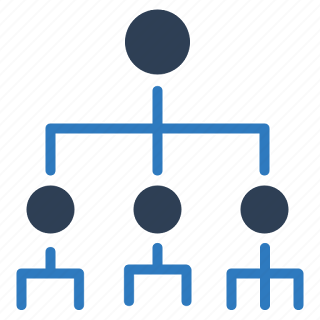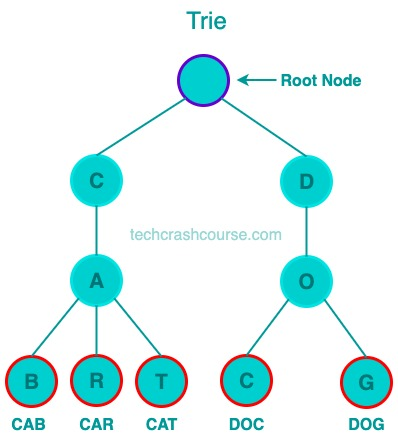# Trie Data Structure# Trie Data Structure

Trie Data Structure is a tree-based data structure that is used to store and retrieve strings efficiently. It is designed to solve problems related to string matching and searching. It is particularly useful in scenarios where we need to perform prefix-based search operations on a large set of strings.

It is a popular data structure in computer science and is used in various applications like search engines, spelling correction, and data compression.## Why do we need Trie Data Structure

Trie Data Structure provides an efficient way to store and search for strings. Unlike other data structures, such as Hash Tables or Arrays, Trie can perform string searches in O(m) time, where m is the length of the search string. This makes it an ideal choice for applications that require efficient string searches.

### Advantages of Trie over Hash Table

Hash Tables and Tries are both used for efficient string search and retrieval. However, Tries have some distinct advantages over Hash Tables:
• Tries can perform string search in O(m) time, where m is the length of the search string, whereas Hash Tables have an average time complexity of O(1) for search operations. However, in the worst-case scenario, - Hash Tables can have a time complexity of O(n), where n is the number of elements in the table.

• Tries provide ordered iteration over all keys, whereas Hash Tables do not guarantee any particular order when iterating over keys.

• Tries can perform prefix matching efficiently, which is not possible with Hash Tables.

### Properties of a Trie

• A Trie is a tree-based data structure where each node represents a character in the string.

• The root of the tree represents an empty string.

• Each node contains a character and a flag that indicates whether the node represents the end of a string.

• The children of a node represent the possible characters that can follow the current character in the string.

• Tries can be used for efficient prefix matching and string search.

## Representation of Trie

Trie can be represented using nodes and pointers. Each node in the Trie represents a character in the string and a list of pointers of child nodes. The children of a node represent the possible characters that can follow the current character in the string.

## How Trie Data Structure Work

To understand how a Trie works, let's consider an example. Suppose we want to store a set of strings { "car", "cat", "cart", "ball", "bat" } in a Trie. We start by creating an empty root node, which represents the empty string.

[Image]

Next, we insert the strings one by one into the Trie. For each string, we start at the root node and traverse the tree according to the characters in the string. If a node corresponding to the character does not exist, we create a new node and attach it to the current node. We also mark the last node of the string as the end of the string.

For example, when we insert the string "car," we start at the root node and traverse the tree according to the characters in the string. We first check if there is a child node with the character 'c.' Since it does not exist, we create a new node with the character 'c' and attach it to the root node. We then repeat the process for the next character 'a' and then 'r.' Finally, we mark the last node as the end of the string.

[Image]

We repeat this process for all the strings in the set, and the resulting Trie would look like:

[Image]

## Basic Operations on Trie Data Structure

### Insertion

To insert a string into a Trie, we start at the root node and traverse the tree according to the characters in the string. If a node corresponding to the character does not exist, we create a new node and attach it to the current node. We also mark the last node of the string as the end of the string. The time complexity for insertion is O(m), where m is the length of the string.

### Search

To search for a string in a Trie, we start at the root node and traverse the tree according to the characters in the string. If we reach the end of the string and the last node is marked as the end of the string, the string exists in the Trie. The time complexity for search is also O(m), where m is the length of the string.

### Deletion

To delete a string from a Trie, we start at the root node and traverse the tree according to the characters in the string. If we reach the end of the string, we mark the last node as not the end of the string. If the node has no children and is not the end of any other string, we can delete it. We continue this process until we reach a node that has children or is the end of another string. The time complexity for deletion is also O(m), where m is the length of the string.

## Trie Data Structure Implementation Program

```#include <stdio.h>
#include <stdlib.h>
#include <stdbool.h>
#include <string.h>

#define ALPHABET_SIZE 26

typedef struct trie_node {
bool is_end_of_word;
struct trie_node* children[ALPHABET_SIZE];
} TrieNode;

TrieNode* create_node() {
TrieNode* node = (TrieNode*)malloc(sizeof(TrieNode));
node->is_end_of_word = false;
for(int i = 0; i < ALPHABET_SIZE; i++) {
node->children[i] = NULL;
}
return node;
}

void insert(TrieNode* root, char* key) {
int n = strlen(key);
TrieNode* node = root;
for(int i = 0; i < n; i++) {
int index = key[i] - 'a';
if(node->children[index] == NULL) {
node->children[index] = create_node();
}
node = node->children[index];
}
node->is_end_of_word = true;
}

bool search(TrieNode* root, char* key) {
int n = strlen(key);
TrieNode* node = root;
for(int i = 0; i < n; i++) {
int index = key[i] - 'a';
if(node->children[index] == NULL) {
return false;
}
node = node->children[index];
}
return (node != NULL && node->is_end_of_word);
}

int main() {
// Create the root node of the Trie
TrieNode* root = create_node();

// Insert keys into the Trie
char* keys[] = { "car", "cat", "cart", "bat", "ball" };
int n = sizeof(keys)/sizeof(keys);
for(int i = 0; i < n; i++) {
insert(root, keys[i]);
}

// Search for keys in the Trie
char* searchKeys[] = { "car", "cat", "cart", "ball", "bat", "dog" };
int m = sizeof(searchKeys)/sizeof(searchKeys);
for(int i = 0; i < m; i++) {
if(search(root, searchKeys[i])) {
printf("%s exists in the Trie\n", searchKeys[i]);
} else {
printf("%s does not exist in the Trie\n", searchKeys[i]);
}
}
return 0;
}
```
Output
```car exists in the Trie
cat exists in the Trie
cart exists in the Trie
ball exists in the Trie
bat exists in the Trie
dog does not exist in the Trie
```

In the above program, we first create a root node and insert a set of strings into the Trie using the `insert` function. We then search for a set of strings in the Trie using the `search` function. The output shows whether each string exists in the Trie or not.